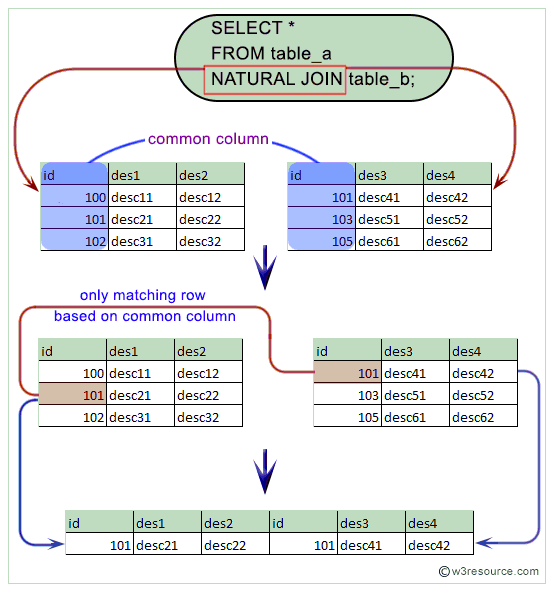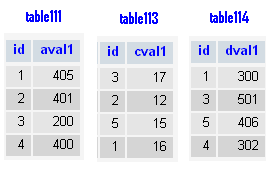# MySQL NATURAL JOIN

## Natural Join

In MySQL, the NATURAL JOIN is such a join that performs the same task as an INNER or LEFT JOIN, in which the ON or USING clause refers to all columns that the tables to be joined have in common.

The MySQL NATURAL JOIN is structured in such a way that, columns with the same name of associate tables will appear once only.

Natural Join: Guidelines:

• The associated tables have one or more pairs of identically named columns.
• The columns must be the same data type.
• Don’t use ON clause in a NATURAL JOIN.

Pictorial presentation of MySQL NATURAL JOIN :MySQL NATURAL JOIN Syntax:

MySQL supports the following JOIN syntaxes for the table_references (A table reference is also known as a join expression.) part of SELECT statements and multiple-table UPDATE and DELETE statements:

```table_references:
escaped_table_reference [, escaped_table_reference] ...

escaped_table_reference:
table_reference
| { OJ table_reference }

table_reference:
table_factor
| join_table

table_factor:
tbl_name [PARTITION (partition_names)]
[[AS] alias] [index_hint_list]
| table_subquery [AS] alias
| ( table_references )

join_table:
table_reference [INNER | CROSS] JOIN table_factor [join_condition]
| table_reference STRAIGHT_JOIN table_factor
| table_reference STRAIGHT_JOIN table_factor ON conditional_expr
| table_reference {LEFT|RIGHT} [OUTER] JOIN table_reference join_condition
| table_reference NATURAL [{LEFT|RIGHT} [OUTER]] JOIN table_factor

join_condition:
ON conditional_expr
| USING (column_list)

index_hint_list:
index_hint [, index_hint] ...

index_hint:
USE {INDEX|KEY}
[FOR {JOIN|ORDER BY|GROUP BY}] ([index_list])
| IGNORE {INDEX|KEY}
[FOR {JOIN|ORDER BY|GROUP BY}] (index_list)
| FORCE {INDEX|KEY}
[FOR {JOIN|ORDER BY|GROUP BY}] (index_list)

index_list:
index_name [, index_name] ...```

Example : MySQL NATURAL JOIN

In the following example, the id is a common column for both the table and matched rows based on that common column from both the table have appeared.

Code:

``````SELECT id,aval1,cval1
FROM table111
NATURAL JOIN table113;
```
```

The INNER JOIN using ON clause do the same job. Here is the following -

Code:

``````SELECT table1111.id,table111.aval1,table113.cval1
FROM table111
INNER JOIN table113
ON table111.id=table113.id;
```
```

Sample tables:Sample Output:

```mysql> SELECT id,aval1,cval1
-> FROM table111
-> NATURAL JOIN table113;
+------+-------+-------+
| id   | aval1 | CVAL1 |
+------+-------+-------+
|    3 |   200 |    17 |
|    2 |   401 |    12 |
|    1 |   405 |    16 |
+------+-------+-------+
3 rows in set (0.00 sec)
```

Example : MySQL NATURAL JOIN with WHERE clause

Code:

``````SELECT id,aval1,cval1
FROM table111
NATURAL JOIN table113
WHERE table111.aval1>200;
```
```

Sample Output:

```mysql> SELECT id,aval1,cval1
-> FROM table111
-> NATURAL JOIN table113
-> WHERE table111.aval1>200;
+------+-------+-------+
| id   | aval1 | CVAL1 |
+------+-------+-------+
|    2 |   401 |    12 |
|    1 |   405 |    16 |
+------+-------+-------+
2 rows in set (0.00 sec)
```

Code:

``````SELECT id,aval1,cval1
FROM table111
NATURAL JOIN table113
natural join table114
WHERE table111.aval1>200;
```
```

Sample Output:

```mysql> SELECT id,aval1,cval1
-> FROM table111
-> NATURAL JOIN table113
-> natural join table114
-> WHERE table111.aval1>200;
+------+-------+-------+
| id   | aval1 | CVAL1 |
+------+-------+-------+
|    1 |   405 |    16 |
+------+-------+-------+
1 row in set (0.05 sec)
```

Key points to remember

Click on the following to get the slides presentation -NATURAL JOINS: SQL and other Relational Databases

Previous: CROSS JOIN
Next: MySQL Subqueries

﻿